# JEE Main 2020 Question Paper with Solution (Jan 6th second shift)

National test agency (NTA) conducted paper 2 of JEE Main (joint entrance exam main) for B. Arch. and B. Planning on 6th January 2020 in two shifts. As per our analysis of 2nd shift paper, it was lengthy and of moderate to hard level. Most of the questions asked in JEE Main 6th January 2020 second shift were from Calculus. We have curated memory based questions of today’s paper and their solutions for you. These questions asked in the second shift of the 6th January JEE Main 2020 paper.

The following are the questions and solutions of 6th January second JEE Main January 2020

Question 1. Let a, b દ R and a>). If the tangent at the point(2,2) to the circle  touches the parabola , then b-a is equal to:

Solution

Slope of tangent to the equation  at point (2,2) is

2x+2yy'=0, put x = 2, y = 2

y’ = -1 (slope=m)

Equation of slope to the parabola in slope form is

Put m = -1 and x = 2, y = 2

We get b-a = 4

Question 2. If the total number of ways in which 8-digit number can be formed by using all the digits 0,1,2,3,4,5,7,9 such that no two even digits appear together in (5!)k, then k is equal to:

Solution:

Digit  0,1,2,3,4,5,7,9.

Odd = 1, 3, 5, 7, 9

_O_O_O_O_O_

Five odd (O) places can be filled by digits  1, 3, 5, 7, 9 in 5! Ways

Now, case 1 when first place is with digit  2

5  place can be filled with the digit 0 and 4 (_) in ways

Now, case 2 when first place is with digit  4

5  place can be filled with the digit 0 and 2 (_) in ways

Total ways (5!)(2 x)

k = 20

Question 3.

Let   and b is real constant. If gof is continuous at x = -1, then b is equal to

Solution:

For continuity at x = -1,

LHL of g(f(x)) = RHL of g(f(x))

b = 5

Question 4. If the line joining the points (-1,2,5) and (3,4,-10) intersects the xy-plane at the point(x,y,z), the  is equal to?

Solution:

Question 5. If  , where c ia s constant of integration, then   is equal to:

Solution:

Use the concept

Question 6. If for some c<0, the quadratic equation,  has two distinct real roots, , then the value of the determinant  is:

Solution:

For two distinct real roots

As Sum of the roots =

So

And the product of the roots =

So

And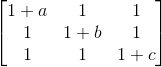=== 3abc=

Question 7.

If the volume of parallelepiped whose coterminous edges are

is 35

1. -10

2. 12

3. 22

4. -14

Solution: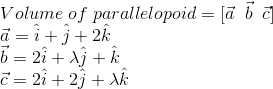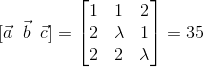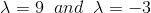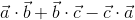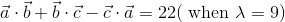Question 8. Which of the following is not equivalent to ?

Solution:

Draw truth table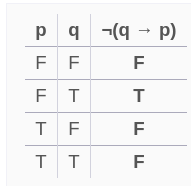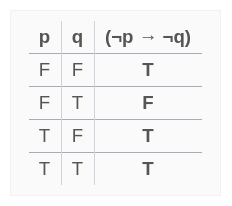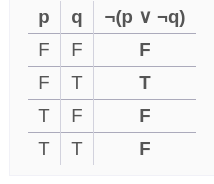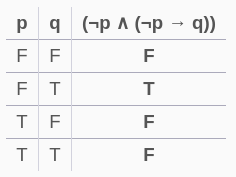Correct option (D)

Question 9. If x = x(y) is the solution of the differential equation, , with , then x is equal to:

Solution:

Question 10. If A={1,2,3,4}, then the number of functions on the set A, which are not one-one, is:

1. 240

2. 248

3. 232

4. 256

Solution:

Total number of functions =

Total number of one-one function

the number of functions on the set A, which are not one-one, is 256 - 24 = 232

Question 11. The function :

1. Decreasing in

Solution

So

So

From the options, we can see that,

from  the f(x) must be increased to reach maxima.

and from it must begin increasing since at   it is minima.

Question 12. A ray of light is projected from the origin at angle of 60o with the positive direction of x - axis towards the line, y = 2, which gets reflected from the point (∝, 2). Then the distance of the reflected ray of light from the point (2,2) is:

1.

2.

3.

4.

Solution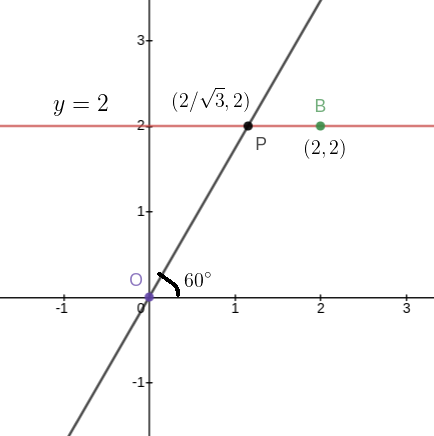Distance PB

Question 13.

Solution-

A=\left[\begin{array}{cc}{x} & {2 y} \\ {-1} & {y}\end{array}\right]

and A^{T}=\left[\begin{array}{cc}{x} & {-1} \\ {2 y} & {y}\end{array}\right]

So , \begin{aligned} A A^{T} &=\left[\begin{array}{cc}{x} & {2 y} \\ {-1} & {y}\end{array}\right]\left[\begin{array}{cc}{x} & {-1} \\ {2 y} & {y}\end{array}\right] \\ \Rightarrow A A^{T}=\left[\begin{array}{cc}{x^{2}+4 y^{2}} & {-x+2 y^{2}} \\ {-x+2 y^{2}} & {1+y^{2}}\end{array}\right]=\left[\begin{array}{cc}{1} & {0} \\ {0} & {\alpha}\end{array}\right] \end{aligned}

This gives us

\begin{aligned} x &=2 y^{2} \ldots(1) \\ x^{2}+4 y^{2} &=1 \Rightarrow x^{2}+4 y^{2}-1=0 \ldots(2) \\ \text { from }(1) & \text { and }(2) 4 y^{4}+4 y^{2}-1=0 \\ \Rightarrow y^{2}=& \frac{1}{2} \Rightarrow y=\frac{1}{\sqrt{2}} \\ \text { And } 1+y^{2}=& \alpha \Rightarrow 1+\frac{1}{2}=\alpha=\frac{3}{2} \\ \Rightarrow \alpha+y^{2} &=\frac{3}{2}+\frac{1}{2}=2 \end{aligned}

Question 14. If 10sin4∝ + 15cos4∝ = 6,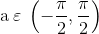, then 27cosec6∝ + 8sec6∝ is equal to:

\text { Question } 14 . \text { lf } 10 \sin 4 \alpha+15 \cos 4 \alpha=6, \quad \text { a } \varepsilon\left(-\frac{\pi}{2}, \frac{\pi}{2}\right), \text { then } 27 \csc 6 \alpha+8 \sec 6 \alpha \text { is equal to: }

\begin{aligned} & 10 \sin ^{4} \alpha+15 \cos ^{4} \alpha=10 \sin ^{4} \alpha+15\left(1-\sin ^{2} \alpha\right) \\=& 10 \sin ^{4} \alpha+15\left(1+\sin ^{4} \alpha-2 \sin ^{2} \alpha\right) \\=& 25 \sin ^{4} \alpha+15-30 \sin ^{2} \alpha \end{aligned}

so given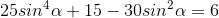; 25 \sin ^{4} \alpha+15-30 \sin ^{2} \alpha=6

So let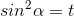; \sin ^{2} \alpha=t

So

\begin{aligned} & 25 t^{2}-30 t+9=0 \\ \Rightarrow & 25 t^{2}-15 t-15 t+9=0 \\ \Rightarrow & 5 t(5 t-3)-3(5 t-3) \\ \Rightarrow & t=\frac{3}{5}=\sin ^{2} \alpha \Rightarrow \cos ^{2} \alpha=1-\sin ^{2} \alpha=1-\frac{3}{5}=\frac{2}{5} \\ \Rightarrow & \csc ^{2} \alpha=\frac{5}{3} \text { and } \sec ^{2} \alpha=\frac{5}{2} \end{aligned}

So

27 \csc ^{6} \alpha+8 \sec ^{6} \alpha=27 *\left(\frac{5}{3}\right)^{3}+8 *\left(\frac{5}{2}\right)^{3}=5^{3}+5^{3}=250

Question 15. The area (in sq.units) of the region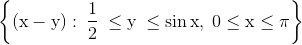is:

\text { Question 15. The area (in sq.units) of the region }\left\{(\mathrm{x}-\mathrm{y}): \frac{1}{2} \leq \mathrm{y} \leq \sin \mathrm{x}, 0 \leq \mathrm{x} \leq \pi\right\}_{\mathrm{is:}}

1.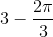; 3-\frac{2 \pi}{3}

2.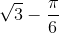; \sqrt{3}-\frac{\pi}{6}

3.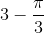; 3-\frac{\pi}{3}

4.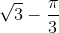; \sqrt{3}-\frac{\pi}{3}

Solution: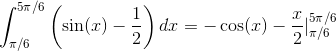=Question 16. The sum of the values of x satisfying the equation, ,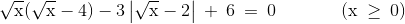, is: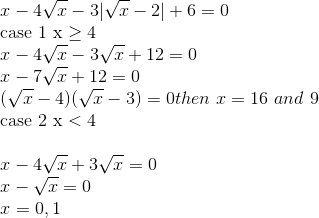Total sum = 16 + 9 + 1 =26

Question 17. Let Sn denote the sum of the first n terms of an A.P, a1, a2, a3,......an. If a5 + a9 = 1 and S9 = 6, then which of the following is not true?

1. a6 + a8 = 1

2. S6 = 19/2

3. S13 = 13/2

4. a13 = 0

Solution: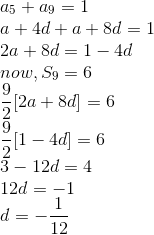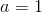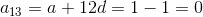Question 18. If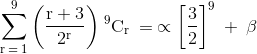, then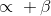is equal to:

1. 9

2. 3

3. 6

4. 2

Solution:

(1+x)n=nC0+nC1x+nCx2+..........................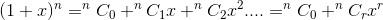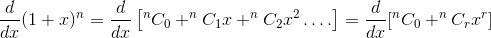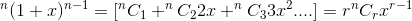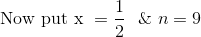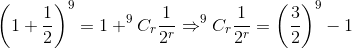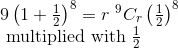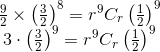We get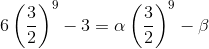From here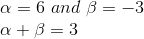Question 19.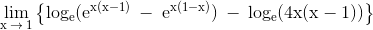is equal to:

1. -2 loge 2

2. 1

3. 1 - loge 2

4. -loge 2

Solution: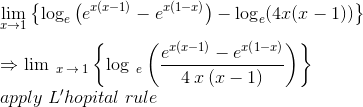= -loge 2

Question 20. Let a function, f:(-1,3)⟶R be defined as f(x) = min{x[x], |x[x]-2| + 2}, where {x} denotes the greatest integer ≤ x, then f is:

Solution: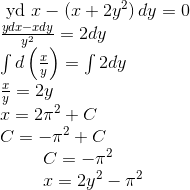Question 21. Let the ellipse,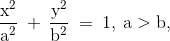pass through the point (2,3) and have eccentricity equal to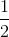. Then, the equation of the normal to the ellipse at (2,3) is:

1. 2y - x = 4

2. 2x - y = 1

3. 3x - 2y = 0

4. 3x - y =3

Solution: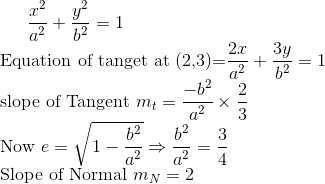Only B option has slope 2

Question 22. A bag contains 6 red balls and 10 green balls. 3 balls are drawn from it one by one randomly without replacement. If the third drawn ball is red, then the probability that the first 2 drawn balls are green is:

1. 3/7

2. 9/49

3. 9/56

Solution: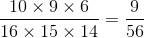Question 23. If a variable plane in 3-D space moves in such a way that the sum of its reciprocal of intercepts on the x and y-axis exceeds, the reciprocal of its intercept on the z-axis by 2, then all such planes will pass through the point:

1.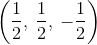2.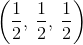3.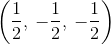4.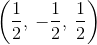Solution: Correct answer is A option

Question 24. Let the data 4, 10, x, y, 27 be in increasing order. If the median of data is 18 and its mean deviation about mean is 7.6, then the mean of this data is:

1. 17

2. 16

3. 16.5

4. 15.5

Solution:

X will be 18 and after solving y will be 18.8 and mean will be 15.5

Correct option D part

Question 25. If m be the latest value of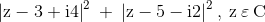attained at z = zo, then the ordered pair (|zo|, m) is equal to:

1.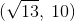2.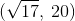3.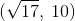4.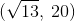Solution:

Correct option is 1st option